How to Use Solver in Excel (Solving Linear Programming Problems)!

The solver tool is used to find an optimum value (either a maximum or minimum depending on the example) for a formula in one cell, by changing decision variables. While solving linear programming problems the solver tool is essential. By reading this article you will get to know how to use solver in Excel.

In order to deliver a solution, Solver needs a group of cells called the variable cells whose values are adjusted, in order to meet the target in the objective cell (the cell which will ultimately contain the maximum or minimum value). These variable cells are subject to constraint criteria.

How to Add Solver to Excel

Although the Solver Add-Ins option is available for all the versions of Excel beginning from 2003, by default it is not enabled. You have to enable it. The procedure for enabling it is given below.

• Click on to the File option on top and then press on to the
• In the Excel Options dialogue box press on to the Add-Ins and there, on to the Manage option select the Excel Add-Ins and press Go.• In the Add-Ins dialogue box select the Solver Add-in and press OK.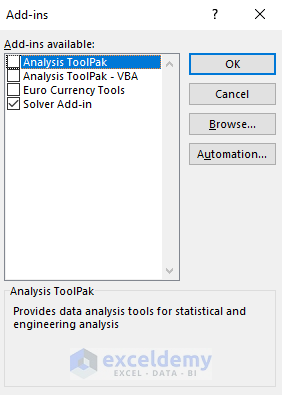• After this, you will see the Solver option is attached to your Excel Data option on the Analyze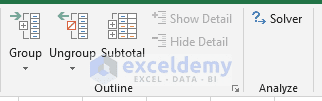Use of Excel Solver for Solving Linear Programming Problems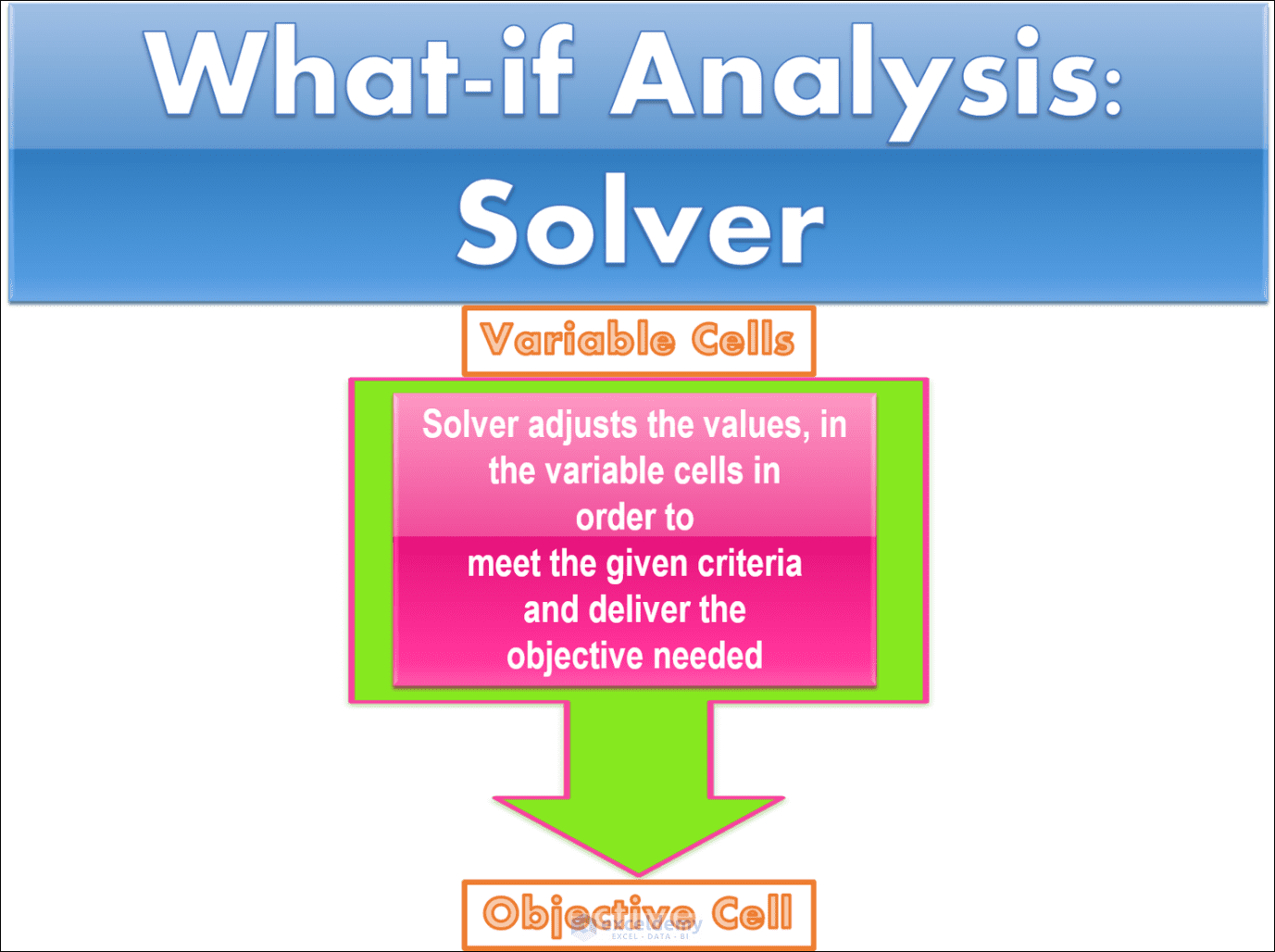Let`s work on an example where we are going to use the solver to determine the maximum reduction in insect growth and viability that can be achieved. The amounts of liquid biopesticide cannot exceed 4000 ml. For the mixture of neem solution at least 5500 ml of the product must be ordered. In addition, 4700 ml of the starch solution must be ordered to fulfill an existing order quota. Also, the overall addition of the three mixtures working in tandem, cannot exceed 14000 ml. The source data for performing solver is given below.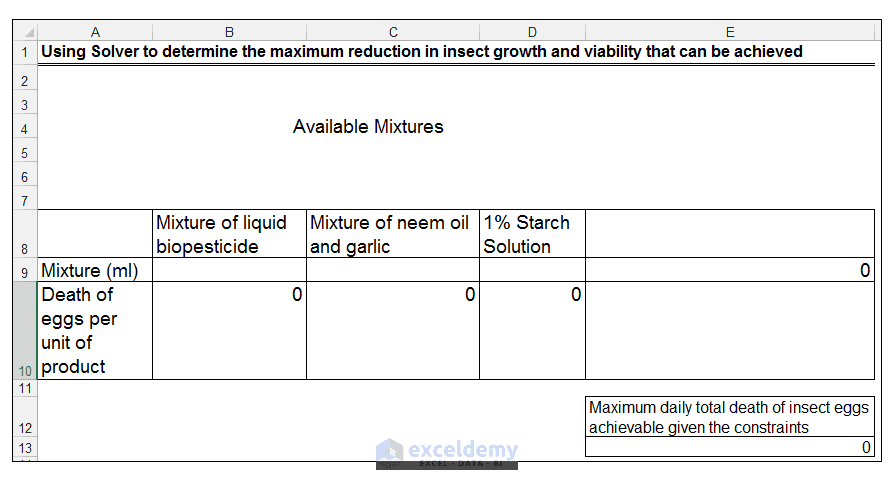1) The variable cells, Solver is going to adjust in order to meet the target is the range B9: D9. The cell range B10: D10 denotes the death of eggs per unit of product. The formula in B10 is 0.6* B9. This formula calculates the estimated death of eggs, based on the level of effectiveness of the mixture of liquid biopesticide. The formula in C10 is 0.10*C9. This formula calculates the estimated death of eggs based on the level of effectiveness of the mixture of neem oil and garlic. The formula in D10 is 0.1 * D9. This formula calculates the estimated death of eggs, based on the level of effectiveness of the starch solution. The total maximum amount of achievable insect egg death is calculated in E13, using the formula =SUM(B10: D10). The value in cell E9 is the sum of all the products used on the farm. In other words, E9 contains the formula =SUM(B9: D9). This is highlighted below.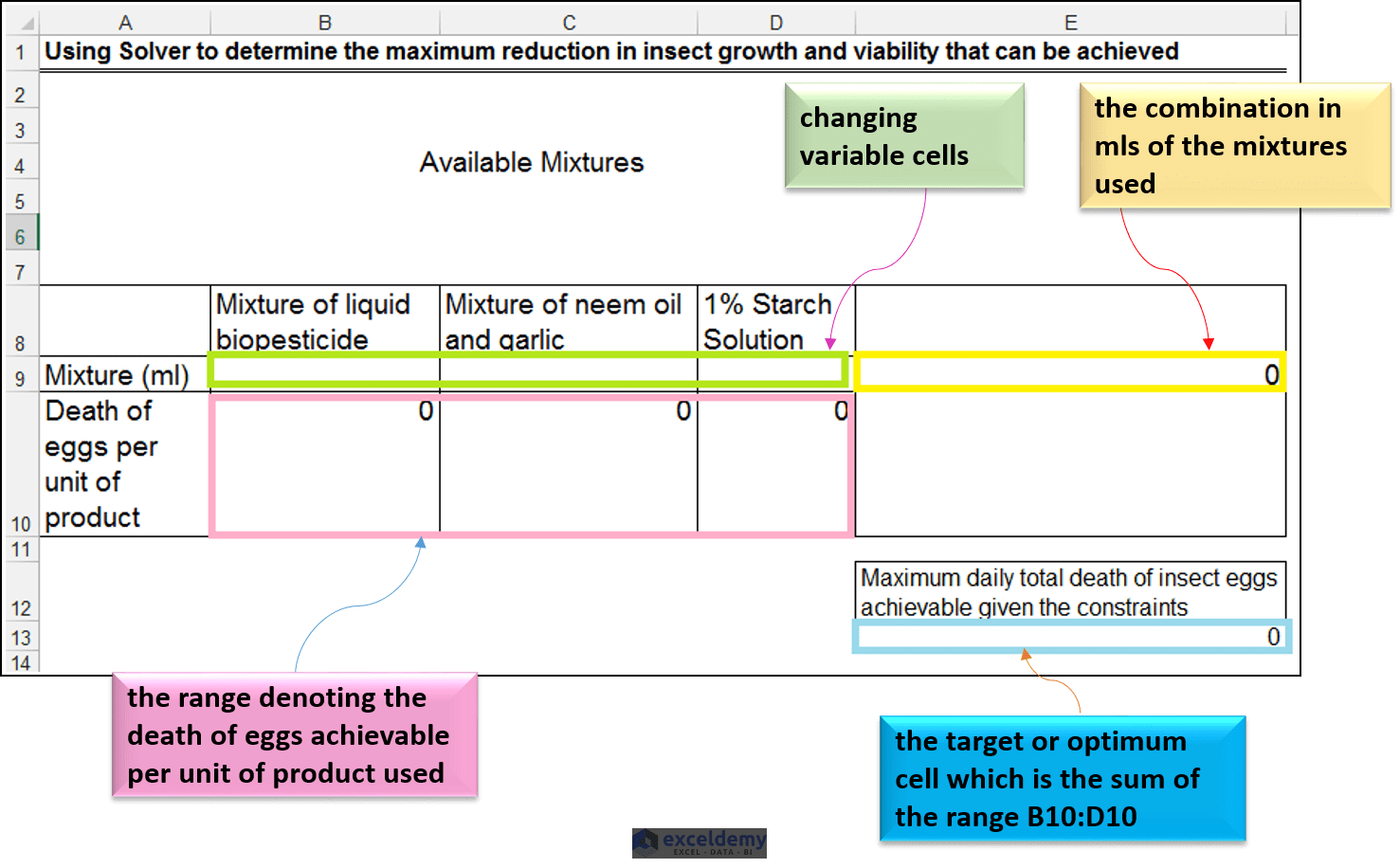2) The constraints are the following:

• The mixture of liquid biopesticide cannot exceed 4000 ml.
• The mixture of neem oil and garlic must be at least 5500 ml.
• The starch solution mixture must be equal to 4700 ml to fulfill an existing order.
• The overall combination of the three mixtures must not exceed 14000 ml daily.

3) Firstly, we go to the Data tab > Analysis group > Solver as shown below.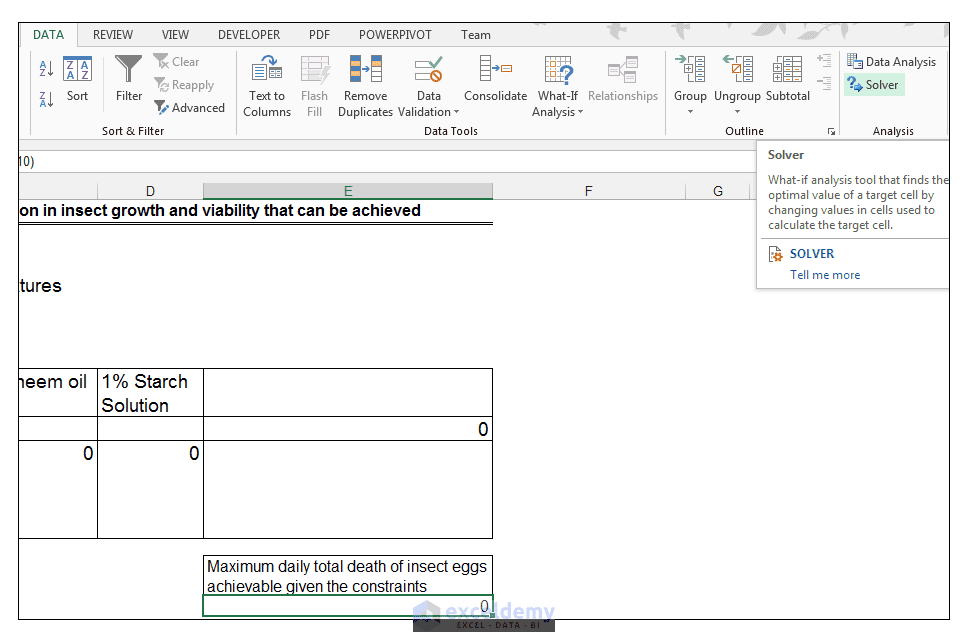4) For our Solver Parameters, we set the objective cell as \$E\$13 and we choose to the max. This makes sense from a logical perspective since we would like to obtain the maximum insect egg cell death, possible.  We select the range \$B\$9:\$D\$9 as our Changing Variable Cells. This is the range we want Solver to adjust, in order to meet our target. For solving method make sure Simplex LP is selected as shown below.5) We are now going to add constraints based on the limitations or criteria the farmer has. So, we click on Add.

Read More: Optimizing an investment portfolio using Excel Solver

6) The first constraint we are going to add is to ensure that the mixture of liquid biopesticide does not exceed 4000 ml. So, we set \$B\$9 <= 4000, in the Add Constraint dialog box. We click Add to set this constraint.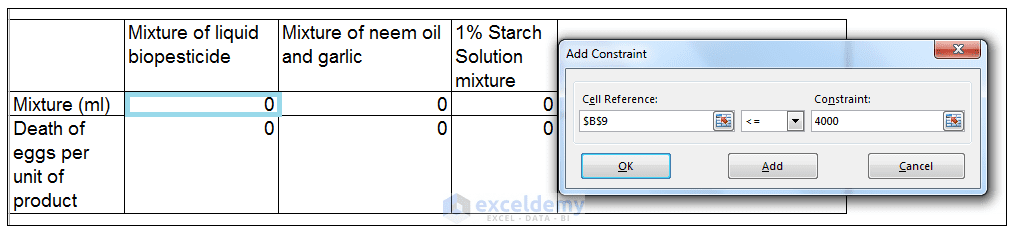7) The next constraint we need to set is to ensure that the mixture of neem oil and garlic is at least 5500 ml. So we set \$C\$9 >= 5500 as shown. We click Add to set this constraint.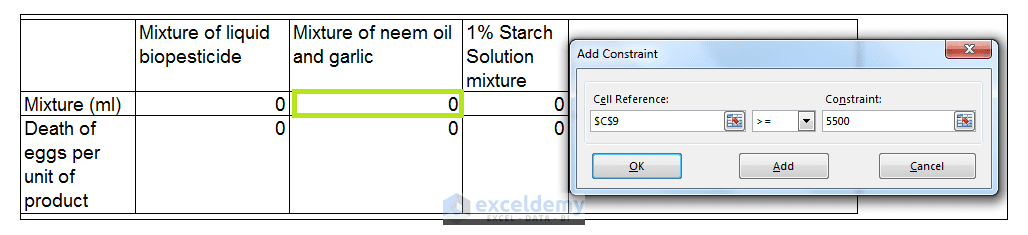8) The next constraint we need to set is to ensure that 4700 ml of the starch solution is ordered in order to fulfill an existing order quota. So we set cell \$D\$9 = 4700.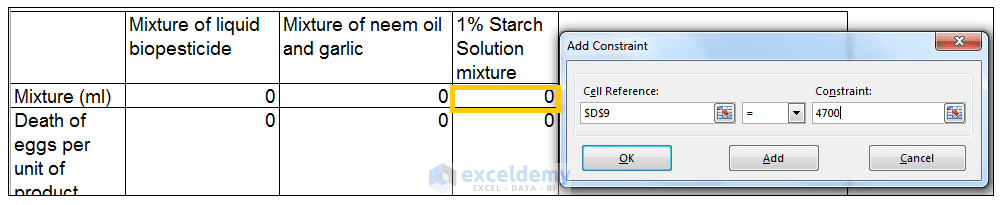9) The last constraint we have to set is to ensure that the total volume of all three products does not exceed 14000 ml. So we set cell \$E\$9 <= 14000 ml as shown. We then click Ok.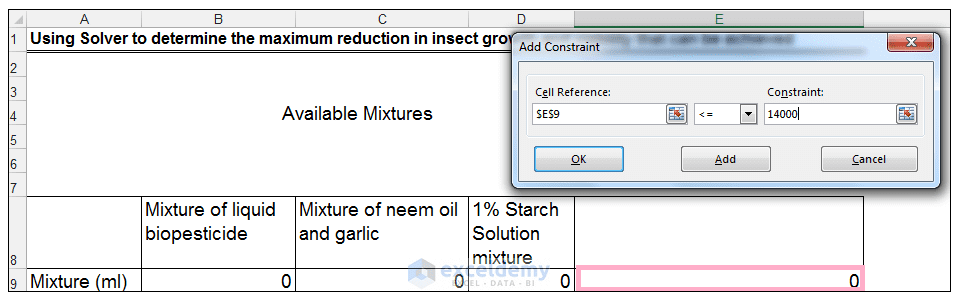10) The parameters are shown below, we then click Solve.11) The Solver Results dialog box should pop up stating that Solver has found a solution. We can either choose to accept the solution or to restore the original values. In this case, we will accept the solution.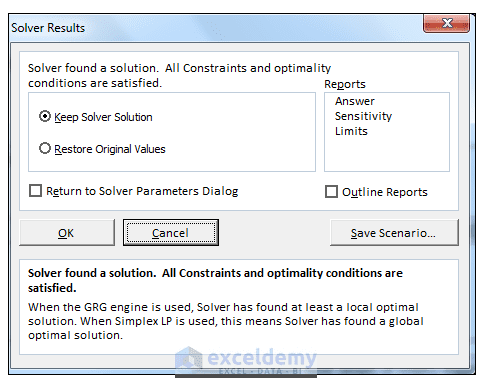12) The solution, Solver has calculated is shown below.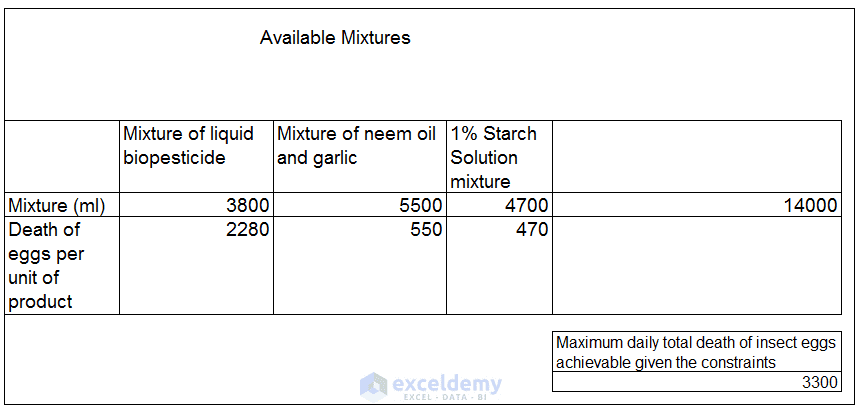13) We can conclude that in order to produce a maximum value of 3300 non-viable eggs given the constraints or limitations we have to adhere too, 3800 ml of liquid biopesticide, 5500 ml of neem oil mixture and 4700 ml of starch solution mixture is needed.

More Excel Solver Example Problems

In our blog, we have many articles from which you will get a clear idea of how to use solver in excel. In the following links, you will find many examples of Excel Solver.

Deal with Sequencing Problems Using Excel Solver!

Financial Planning with Excel Solver [2 Case Studies]

Minimizing shipping costs in Excel using Solver

The algorithm of Excel Solver

Excel Solver has many different algorithms for finding the solution of different complex linear and non-linear problems. We can choose any of the following method in the Select a Solving Method box.

• GRG Nonlinear: For the smooth nonlinear problems Generalized Reduced Gradient Nonlinear algorithm is used where the data will contain minimum one constraint which will be a smooth nonlinear function.
• LP Simplex: The LP Simplex Solving method is used for solving a problem of Linear Programming which is characterized by linear relationships. With the linear relationship among the data, the linear programming tends to achieve the desired goal such as minimum loss, maximum profit etc.
• Evolutionary: It is the most difficult optimization problem where you can not determine the characteristics of the function. The direction of this kind of function can be increasing or decreasing. This is because the functions are either discontinuous or nonsmooth.

Conclusion

Solver is a useful tool for advanced what-if analysis. When using Solver, one aims to find an optimum value, while still working within the context of environmental constraints.